Odkaz jsme Vám odeslali na email.

Nepodařilo se nám odeslat odkaz na váš email. Zkontrolujte, prosím, Váš email.

# Online nápověda

Stromeček
Nastavení
Produkt:
Program:
Jazyk:

## Modified Cam-Clay Model (MCC)

The MCC model was originally developed for triaxial load conditions. Experimental measurements on soft clays provided the background for the development of the constitutive model expressing the variation of void ratio e (volumetric strain εv) as a function of the logarithm of the effective mean stress σmeff, as evident from the following Figure. Both graphs are related as follows: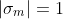κ - slope of swelling line [-] λ - Slope of NCL (normal consolidation line) [-] e - current void ratio [-]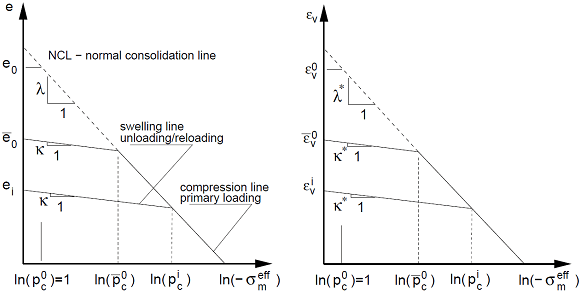Material response during isotropic consolidation (constitutive model)

The graph consists of a normal consolidation line (NCL) and a set of swelling lines. On the first load the virgin soil moves down the NCL. Next, suppose that the soil was consolidated to a certain level of stress, which is termed the preconsolidation pressure pc, and subsequently unloaded up the current swelling line. Then, upon reloading the soil initially moves down the swelling line until reaching the stress state given by the parameter pc, which existed prior to the unloading.  At this point the soil begins to move again down the normal consolidation line (primary loading - compression line).

Parameters κ and λ can be estimated from the following expressions: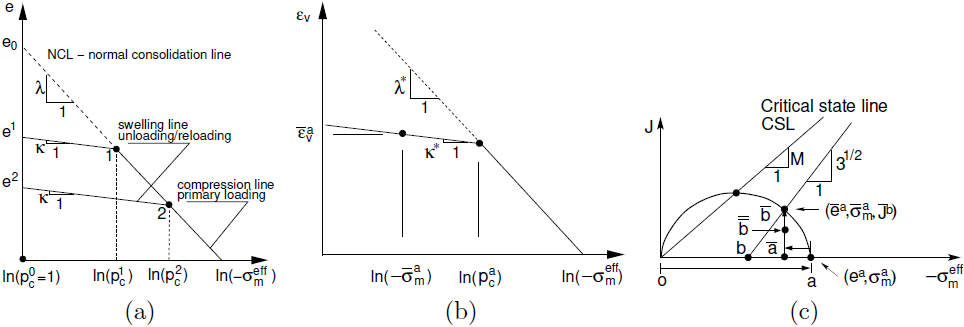where: Cc - one-dimensional compression index Cs - one-dimensional swelling index

These parameters follow from a simple oedometric test.

The yield surface is smooth without the possibility of evolution of tensile stresses. The MCC model allows, unlike the first group of models, a direct modeling of strain hardening or softening for normally consolidated or overconsolidated soils, a nonlinear dependence of the volumetric strain on the effective mean stress and limit conditions of ideal plasticity. When using the MCC model the soil loaded in shear can be plastically deformed without collapse (points 1,2 for hardening, point 2 for softening) until reaching the critical state (points 3 and 2 for hardening and softening, respectively). The soil deforms further in shear under the assumption of ideal plasticity without the change of e and σmeff. Upon unloading, a linear response of soil is assumed.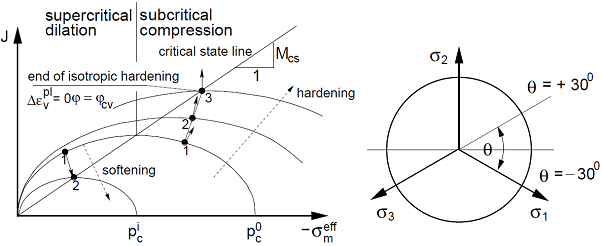Projection of yield function into meridial and deviatoric planes

Evolution of the yield surface (hardening/softening) is driven by the current preconsolidation pressure pc: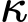where: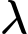- current preconsolidation pressure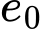- increment of volumetric plastic strain

Apart from parameters κ and λ, the self-weight and the Poisson's ratio, the MCC model requires specifying the following three parameters:

 Mcs - slope of the critical state line [-] OCR - overconsolidation ratio [-] e0 - initial void ratio [-]

Reliable initialization of the model is described in section "Numerical implementation of MCC and GCC models".

The slope of the critical state line Mcs can be determined from the expression: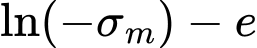, for triaxial compression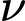, for triaxial extension

where φcv is the angle of internal friction for constant volume corresponding to the critical state.